<![CDATA[Creative morphometrics]]> 2015-01-19T20:57:22+01:00 http://paulidealiste.github.io/ Octopress <![CDATA[Efficient Outlines Around Points in R]]> 2015-01-18T10:25:19+01:00 http://paulidealiste.github.io/blog/2015/01/18/efficient-outlines-around-points-in-r Convex hull is one of the most widely used techniques for finding the minimum enclosing shape around a set of points in a plane, but for certain tasks it is necessery to find such shape which encloses concave parts of the outline as well. Convex hulls are also sensitive to localized point groups, far apart from the majority of other points. In these cases, hulls enclose a lot of empty space so that the possible underlining shape is obscured. In geometric morphometrics, outlines around the set of landmarks in the plane can provide the idea of shape, useful for visualization of the underlining shape (i.e. the shape described by these points). Possibly, such outlines may be analysed further, with the usual GM analytic procedures. Alpha shape1 is the generalization of the convex hull for a set of points in the plane which can be used for shape reconstruction, since the frontier of an alpha shape is a linear approximation of the original shape. In R, this technique is implemented in the alphahull library. For this post, randomly generated points-in-the-plane data will be used.

For a set of points, alpha shape is based on the Delaunay triangulation and its corresponding Voronoi diagram, so they are found first, followed by alpha shape and alpha hull.

The value of the parameter alpha is determined with respect to the dimensionality of data points, since it represents the minimal distance for icnlusion or exclusion of the neighbouring points within the alpha shape procedure.

When the value of alpha is selected taking into account the spacing between the points, the enclosing outline can be obtained so that it approximates the most probable shape of the points in the plane (Figure 1, Figure 2).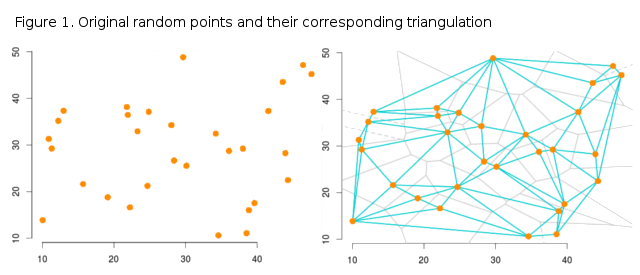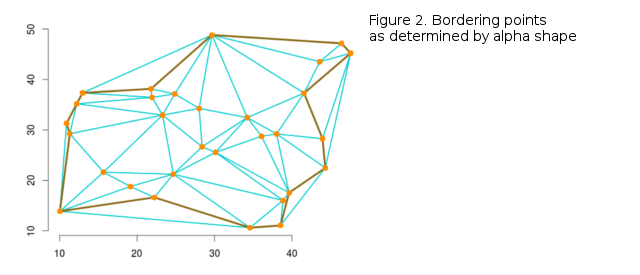1. Edelsbrunner, H., Kirkpatrick, D.G. and R. Seidel. 1983. On the shape of a set of points in the plane. IEEE Transactions on information theory, 29: 551-559.

]]>
<![CDATA[PLS Model for Temperature in R]]> 2014-09-02T13:01:59+02:00 http://paulidealiste.github.io/blog/2014/09/02/pls-model-for-temperature-in-r Continuing on the post about the climate data extraction in R, this post demonstrates a simple approach to using PLS (partial least squares) for determining the common covariance patterns between morphology and mean monthly temperature (although the model is likely to be bad). Morphology data can be found here. This dataset only contains PCA scores of individuals for the first two PC axes (Figure 1), extracted from the Fourier descriptors of horn shape. PC1 axis (92%) clearly separates males and females on the basis of respective horn shape.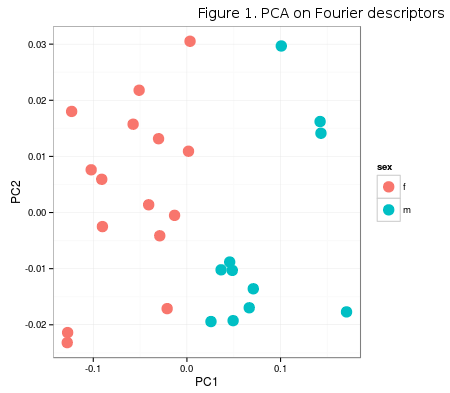Temperature data are extracted using geoTiffs from WorldClim database, as in the mentioned post about climate data extraction. The ready-made dataset of mean monthly temperatures for the above study locality can be found here.

PLS analysis is a useful multivariate technique used for determining the common variation patterns in two blocks of data and is sometimes reffered to as PLS regression. In this post, of all PLS implementations in R, the choice is on the fabulous plsdepot library, developed and maintained by Gaston Sanchez, whose blog/personal page and work in general was a great insipiration for Creative Morphometrics. His approach is very well explained and documented over at his page, so only direct implementation on the data above will be provided here.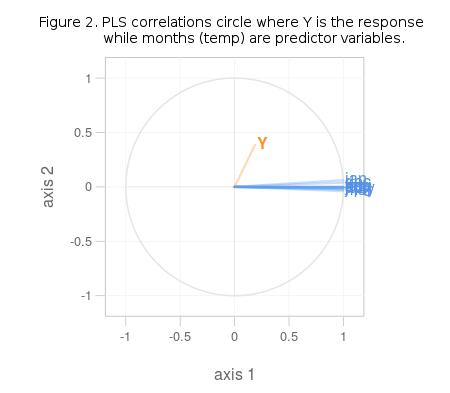It is obvious from Figure 2. that the variables in question share no common variation pattern and are totally unrelated. If the chosen data was better, some of the blue lines (predictors) would run parallel with the orange one (response). If the R2 value for this model is examined (it is a part of climatePLS object – climatePLS\$R2) the unrelatedness of predictors and response in this model gets even more obvious (R2 = 0.035). Predictors in this model are also highly correlated within themselves, which renders them rather useles in prediction, as was stated at the onset.

]]>
<![CDATA[Automated Outlines With openCV in Python]]> 2014-08-05T20:06:18+02:00 http://paulidealiste.github.io/blog/2014/08/05/automated-outlines-with-opencv-in-python Quantification of biological shape often relies on manual extraction of information from a set of digital images or 3D models, like manual landmark placement or outlining the object of interest by hand. With computer vision approach and, especially, with the help of the openCV library, a set of image manipulation tecniques as well as automated feature extractions are facilitated to a large extent. Since there are excellent python bindings for this library it is easy to test ideas for image analysis and object extraction in the context of geometric morphometrics.

This post will serve as a general introduction to openCV in python, and will continue on the earlier posts about the outline extraction from digital photos usual in GM research. The sample image can be obtained here.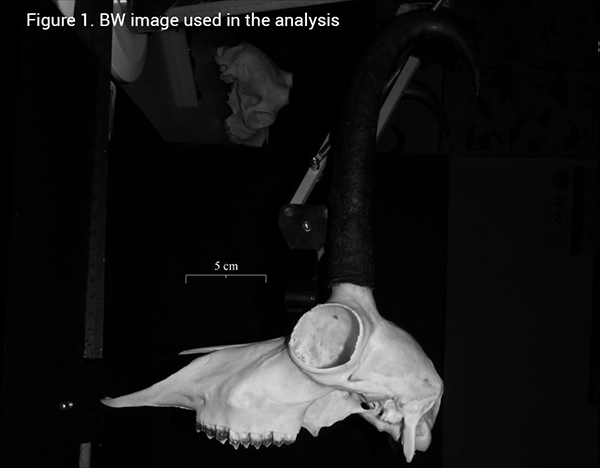This image is suitable for automated online extraction of the skull, since there is a significant ammount of contrast of the object and the background. The idea is to use thresholding and a bit of erosion around the edges to delimit the skull from the rest of the picture and then apply automated contour extractions.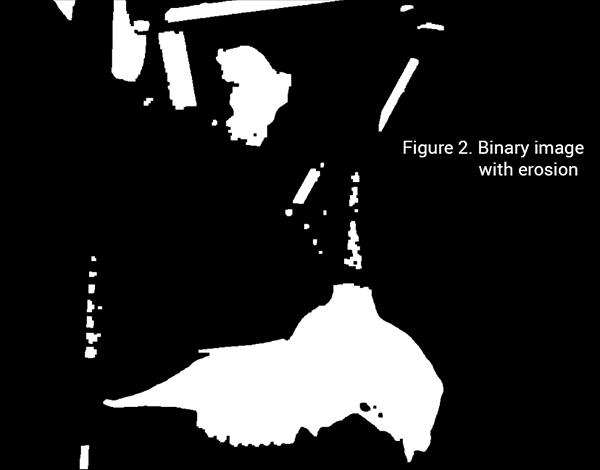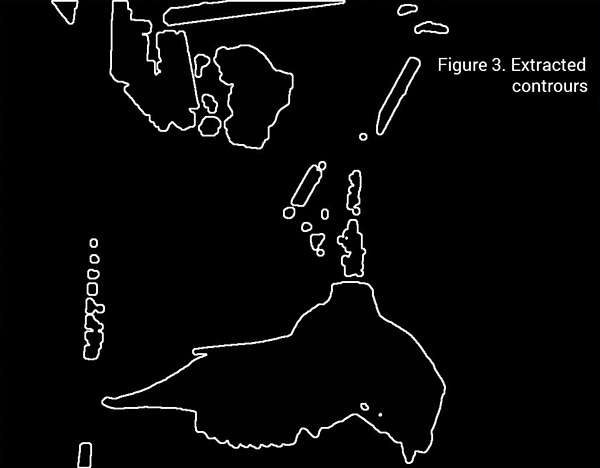Finally, since other objects of no interest are also outlined in Figure 3, the skull outline must be identified in the array list contours. This can be done by a for loop, and the openCV method for calculating outline areas, as the skull outline is the longest continual outline in the image.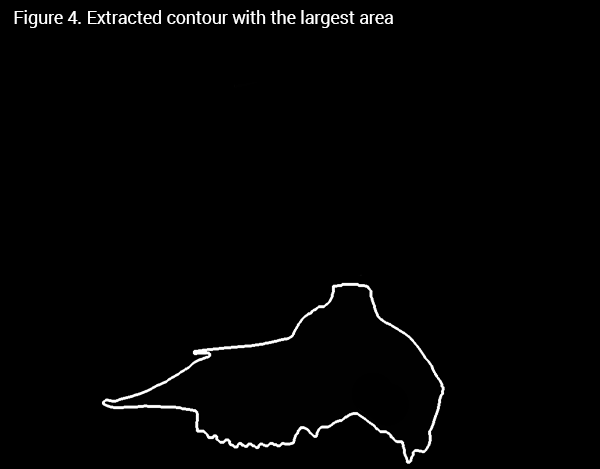The extracted contour is a numpy array with xy coordinates of contour points in rows, so it can be directly used for sampling landmarks along the outline for fitting the normalized Fourier transform and doing shape analysis further. Of course, the procedure can be easily generalized to work over all images in a dataset, extract the contours with largest areas from each image, and generate a dataset for shape analysis.

]]>
<![CDATA[Python Project and Explore]]> 2014-07-07T17:21:08+02:00 http://paulidealiste.github.io/blog/2014/07/07/python-project-and-explore Since Procrustes superimposition introduces the data into a curved shape space which corresponds to a hyperhemisphere of radius 1 with numerous dimensions (as defined by Rohlf, 19991), usual multivariate statistical methods are not readily applicable to such data. This data must be projected to a flat, Euclidean tangent space, that is in contact with the hyperhemisphere in one point, taken as a mean shape of the whole dataset. This projection can be applied only if the variation in shapes in not overtly large so that their “shadows” on a flat space are not too far apart. In this post, data will be generated as usual and Procrustes procedures will be taken from previous posts, using defined functions centsiz, transScale, mshapr, pProc and pGP.

After Procrustes superimposition, data can be projected orthogonally and stereographically. More common method is the orthogonal projection which minimizes lagre shape differences between landmark configurations. The procedure is based on Rohlf, 1999, suggesting that the matrix of aligned centered preshapes should be multiplied by the mean shape of unit centroid size, subtracted from the identity matrix of comparable dimensionality (Claude, 2008).

Numpy array projCoords holds wide representation of projected data (rows are individuals, while columns are all landmarks, first all x and then y coordinates), ready to be used in PCA or any other multivariate method. In order to illustrate potential grouping in PCA morphospace, since the data is randomly generated, a kmeans clustering will be performed with 3 groups, just to effectively split the data. PCA can be done in python in numerous ways, but in this post a PCA decomposition from scikit-learn package is used.

Since the data is randomly generated, there is not much structured variation in it, as three of the first PC axes describe 14.45%, 13.29% and 12.46% of total sample variability, respectively. On the other hand, kmeans forces the data into three groups, which is useful for demonstration of plotting in matplotlib with 3D axes, and a groupping structure in PCA scatterplots (Figure 1), similar to real-world data.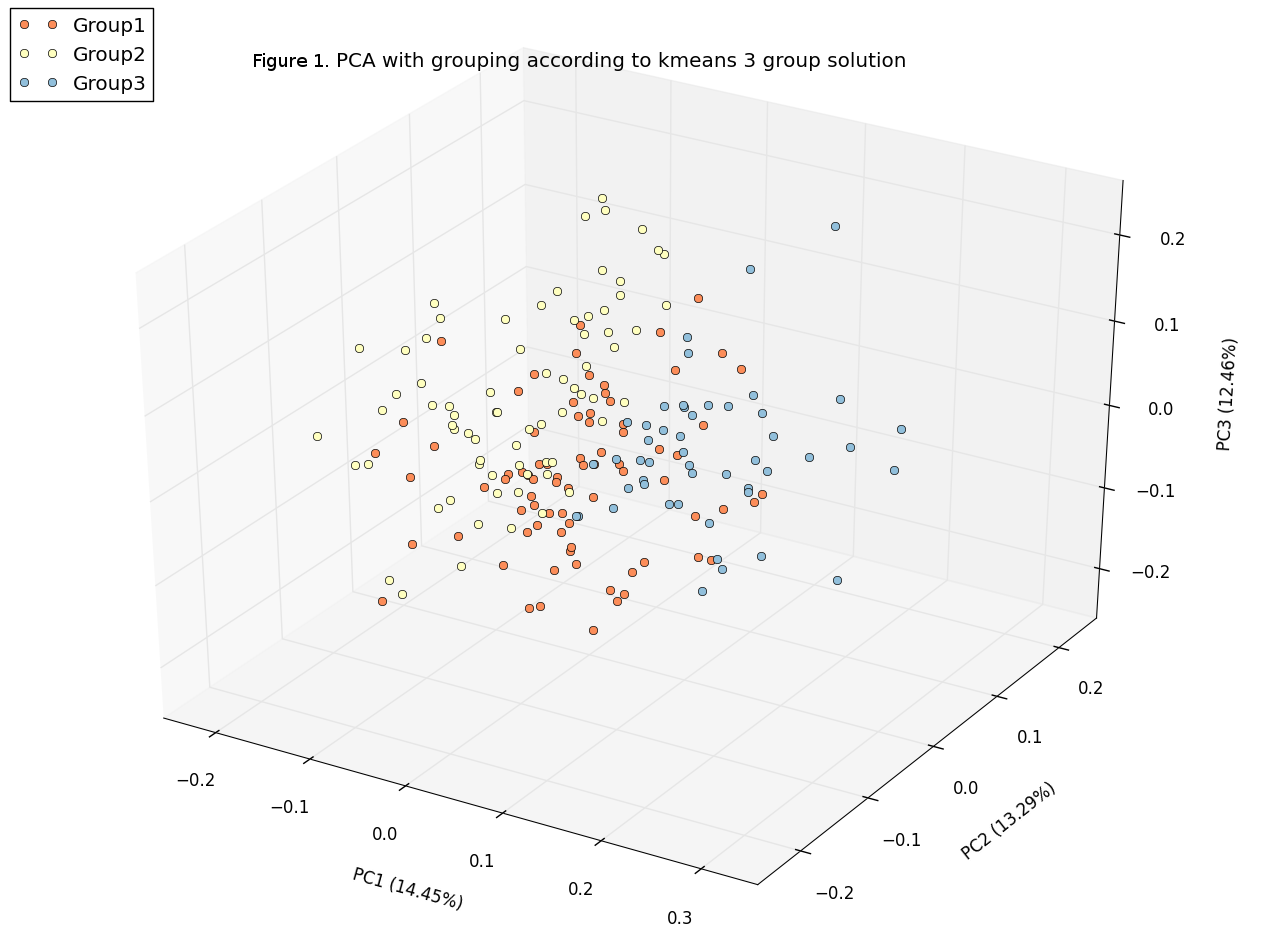1. Rohlf, J.F. 1999. Shape statistics: Procrustes superimpositions and tangent spaces. Journal of Classification 16: 197-223.

]]>
<![CDATA[Simple Angles and Nice Plots]]> 2014-06-09T17:28:06+02:00 http://paulidealiste.github.io/blog/2014/06/09/simple-angles-and-nice-plots Angles were important for some of the most interesting research in morphometrics. The approach of Rao and Suryawanshi, 19981. introduced the angles within the landmark triangulation grids as an alternative to Procrustes superimposition for extracting shape data. This post does not perform the procedures described by Rao (which are ported to R in “Geometric morphometrics in R” by J.Claude, 2008), but instead aims at extracting angle data, from configurations of landmarks, after Procrustes superimposition. The code presented here is motivated by the polarRotator function from some of the previous pyhton-related posts, and it presents landmark data as polar angles. These angles represent the angular deviation of each landmark from the centroid of the respective configuration (Figure 1), so that the variability in their position with respect to xy centroid coordinates, may represent their shape differences. Since superimposition procedure sets all configurations centroids to the origin, they are all 0,0. Dataset used is the same as for the triangulation post.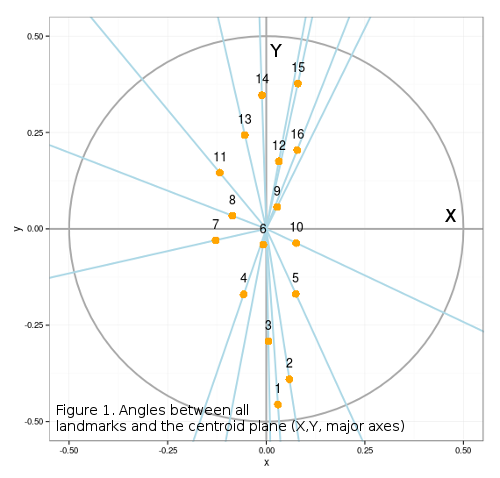ggplot2 has one great geom, polar_angle() that will transform any shape, from Cartesian to polar coordinates. Unfortunately it does not give polar angles as well, but the plot is informative (Figure 2), since landmarks are ordered according to their angular deviation from 0, i.e. the X and Y axes defining the 0.5 centroid-centered ellipse.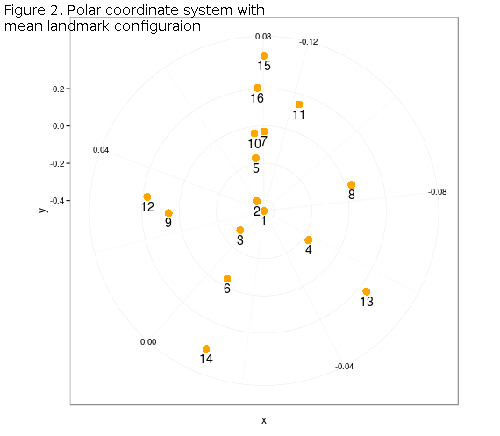Polar angles can be calculated by using the atan2 (arctangent) function, which takes two parameters, x and y and gives one point estimate of the polar angle in radians. This number is really the angle between the positive x-axis of a plane (X from Figure 1) and the point given by its xy coordinates. Since after superimposition the x-axis lies at zero for all landmark configurations, it would be sufficent to use only xy coodinates of landmarks, since centroids are also at 0.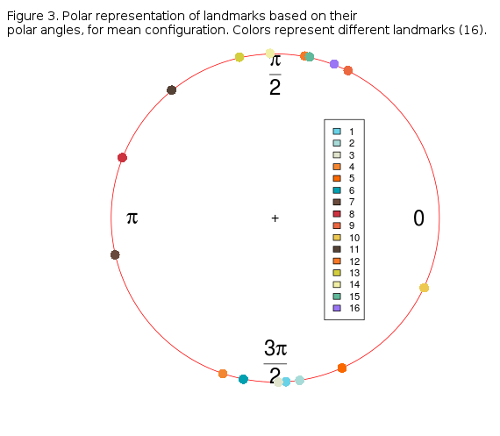Figure 3 shows the distribution of landmarks from along a circular path (0, π). It is obvious that landmarks form two distinct groups, one above, and one below the x-axis, with the exception of landmarks 7, 8 and 10 which are the most parallel to the x-axis. The proportion of the overall shape variability left in these angles must be thouroghly checked, but it is not easy, since angle-data can not be analysed by conventional statistics, but by circular or compositional methods. R provides some libraries for this, circular and CircStats which are both derived from the book of Jammalamadaka and Sengupta, “Topics in circular Statistics” from 2001. If one more individual is added to the circle-plot (Figure 3) it can be can visually inspected how much its coordinates (landmark polar angles) deviate from the sample mean (Figure 4).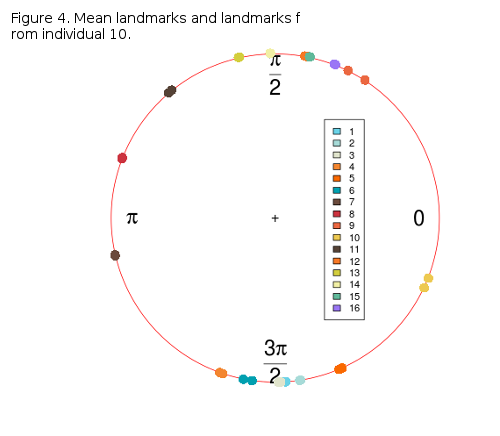Some variablity is present around landmarks 10, 6 and 5, while others are nearly or completely overlapping. The variability may not be large, but it will be checked in future posts, as well as possible PCA with the angular data.

1. Rao C.R. and S. Suryawanshi. 1998. Statistical analysis of shape of objects based on landmark data. PNAS 93: 12132-12136.

]]>
<![CDATA[Complete Procrustes in Python]]> 2014-05-17T20:38:16+02:00 http://paulidealiste.github.io/blog/2014/05/17/complete-procrustes-in-python Procrustes superimposition is the first analytic step in geometric morphometrics and this post shows one possible solution to performing it in Python1, using several functions defined in previous posts. First steps include data generation and definition of functions important for extracting shape variables from randomly generated landmark data. These functions are centsize which calculates centroid size from a numpy array (xy data) and transScale which translates landmark coordinates to the origin of the coordinate system and scales them to unit centroid size. The mshapr function is needed in the main superimposition function since it calculates succesive mean shapes (all configurations), excluding the configuration that is currently being rotated.

Definition of the pProc function follows the same rules as in the previous post, with the robust (and ugly for now) if clause that is only included to allow either pandas DataFrame or a numpy array as the input data (both for configurations and mean shape objects). It also returns only the rotated matrix, landmark configuration (pmat1) and not the mean shape.

Finally, the pGP function, that can perform partial Procrustes superimposition for any number of individuals (landmark configurations) and landmarks. Generated data has 200 individuals and 10 2D landmarks. For now this function will ask such information in the function call, so that mmat1 is pandas DataFrame with x, y, coordinates and individuals columns (generated above), numind is the number of individuals, dim is 2D (3D not yet supported), and numland is the number of landmarks. For clearer understanding of the following code, comments are included where appropriate.

DataFrame tempRot holds the superimposed configurations (shape variables) that can, subsequently, be used in standard GM analyses and further. Of course, graphical display of superimposition results can be very interesting, and in this post only basic plots will be given, while some of the further posts may include more visualizations. Figure 1 shows the original raw-generated data, Figure 2 the spatial relationships between raw and superimposed data, while Figure 3 shows only superimposed data.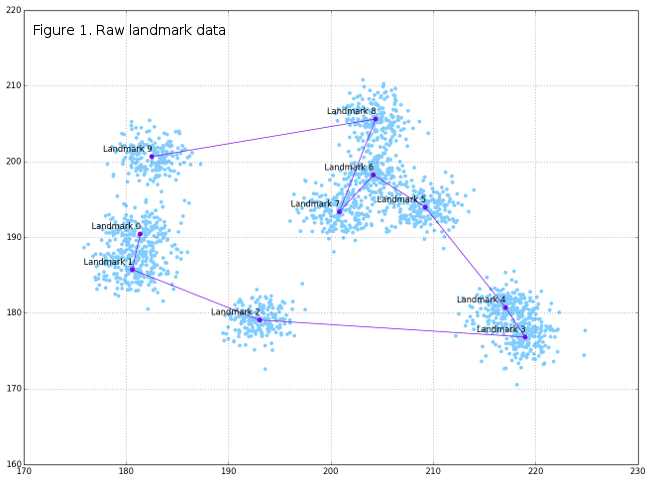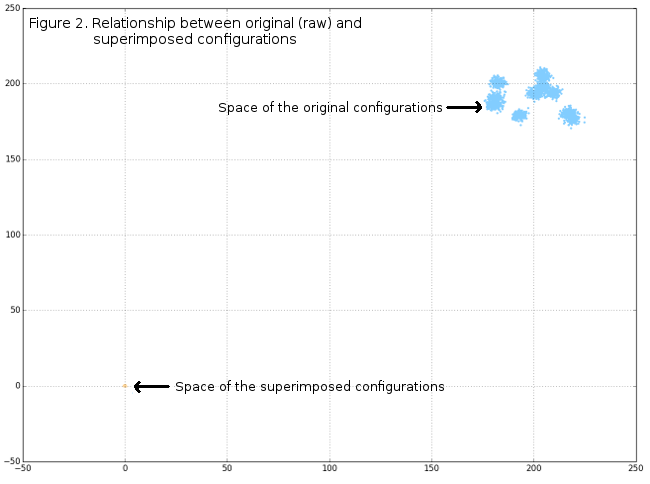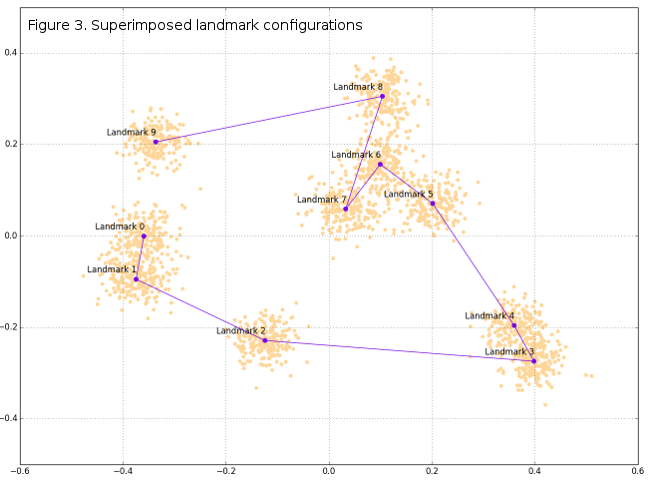1. If using IPython (:)) best way is to paste code with the %paste magic function.

]]>
<![CDATA[Modularity, Graphs and Triangulations]]> 2014-05-03T00:08:25+02:00 http://paulidealiste.github.io/blog/2014/05/03/modularity-graphs-and-triangulations Continuing on the previous post, procedures presented here show the comparison between the usual assesment of modularity in the collection of landmarks (same dataset as before) and the depiction of modular structure through graph theory. Usually, modularity in the mammalian cranium is determined a-priori, according to some of the established hypotheses about the developmental origin of cranial elements. One of the most prevalent hypotheses is the two-module organization, one module comprising of elements originating mainly from the neural crest embryonic tissue (anterior cranium) and one comprising of elements with somitomeric origin (posterior cranium). Following code shows the test of this specific hypothesis using geomorph package functions, and additional usage of deldir for visualization of modularity hypotheses.

Figure 1 shows the theoretical distribution of the RV coefficients, calculated for all posible two-module subdivisions of landmarks, as well as the observed RV value from the hypothesis depicted in Figure 2. Since the observed value is lower than most of the hypothetic values, then it could be said that this modularity hypothesis stands.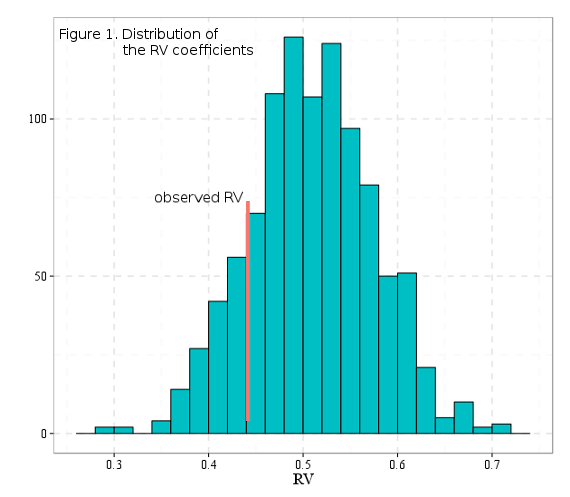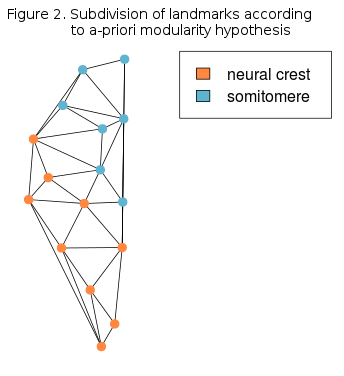The idea for using graph theory representation and analysis of modularity is based on correlation matrix, same one from the previous post. Correlation matrix was obtained through Delaunay triangulation and every vertex (node) in the graph actually represents the 1/3 of the total area of all Delaunay triangles emanating from the corresponding landmark.

Graph based on the correlation matrix can be created and manipulated with the wonderful igraph package. Vertices of this graph will be abovementioned areas, while the edges will represent correlation (edge weights) between areas around each landmark. According to weights, edges can be colored and the strength of thier lines increased, so that the most correlated landmarks wolud be more obvious.

Modularity, or community structure in the graph theory represents the degree of compartmentalization in the graph structure, based on the spatial relationship or the weights of graph edges. Although, a-priori subdivision of graph vertices is possible, and the evaluation of such graph community can be easily done, the advantage of graph theory for modularity is the availability of algorithms for searching the community structure, so it can derive subdivsion a-posteriori, that can be compared to the hypothesis from Figure 1.

Leading eigenvector community algorithm tries to find community structure in the graph by calculating the eigenvector of the modularity matrix for the largest positive eigenvalue and then separating vertices into two communities based on the sign of the corresponding element in the eigenvector (Newman, 20061). This method was preffered over many others because it is closer to usual multivariate methods, such as PCA, that are commonly used to depict variability patterns.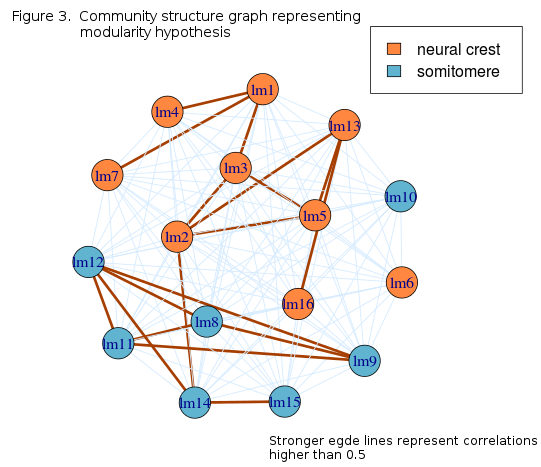Graph community strucure depicts modularity in the landmark configurations accurately, with the exception of lm16, that is positioned on the posterior basicranium. Higher correlations are, on average, present within modules while between modules, only one connection is higher than 0.5, that between lm2 and lm14. Since these landmarks lay on the opposite sides of the cranium, they may encompass the variability in total anterior-posterior length. i.e. cranial size.

1. Newman, M.E.J. 2006. Modularity and community structure in networks. PNAS 103: 8577-8582.

]]>
<![CDATA[Delaunay Triangulation Experiment]]> 2014-04-04T18:09:37+02:00 http://paulidealiste.github.io/blog/2014/04/04/delaunay-triangulation-for-modularity-pt-1 Since landmark data consists of x and y Cartesian coordinates, it would be useful if they could be represented by one summary number, that would preserve the spatial information. This is just a proposal of one possiblity using Delaunay triangulation between landmark configurations, and the average area of all triangles emanating from individual landmarks. Since landmark position within the triangulation grid would influence the shape and size as well as number of triangles around it, the area should preserve enoguh information about the spatial relationships of landmarks. Dataset for this post consists of ventral aspect half configurations from here. Delaunay triangulation will be performed using deldir R package, since it reports the mentioned triangle areas as a part of object summary.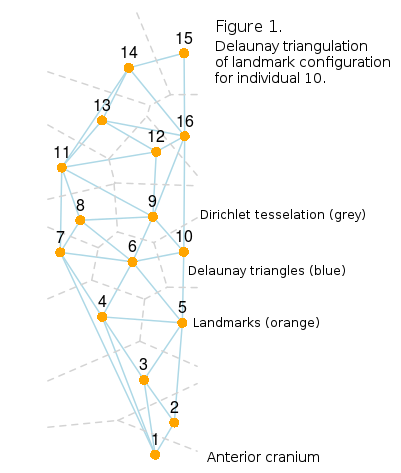Values in the areaCap matrix represent average areas of all triangles emanating from the landmark in question, so its dimensions are 60x16. Correlation matrix (16x16) can be calculated from the areaCap matrix, and it should reflect relative positioning of landmarks through spatial grouping pattern. This can be checked visually using corrplot package visualization of the correlation matrix, which allows direct assesment of emerging patterns in the matrix. Additionally, this package allows reordering of rows and columns according to hierarchical clustering algorhithms and representing the desired number of clusters with rectangles in the correlation plot. This can only be considered as an idea of general modularity, since landmarks from the same cranial region should be correlated more than distant landmarks. But the transformation of xy coordinates to average triangle areas may have introduced non-biological variation or obscured some of the natural variation and these procedures as well as subsequent analyses may be treated only as a fun experiment and visualization tool for now.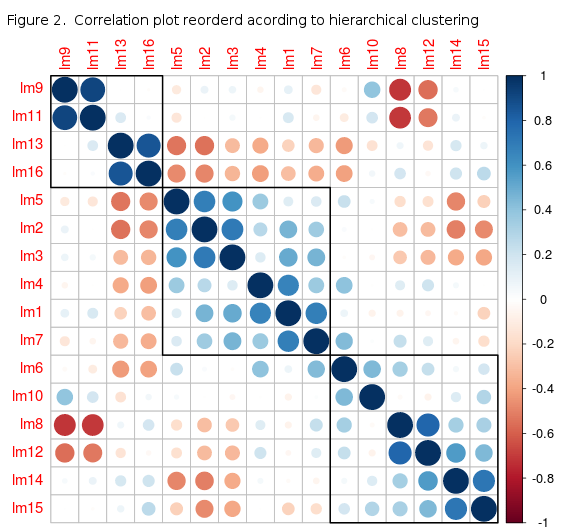Correlation plot with three proposed general landmark groups in the matrix reveals that the correlations are, on average, higher for locally grouped landmaks, especially the anterior ones, from 1 to 7. This can`t be used as a reliablie test for modularity hypothesis, but it can serve as a basis for further analyses based on construcing linked graphs or networks using correlation matrices from landmark data, where the xy coordinates are transformed to one number. Also this can be a useful way of representing modular structure visually, since both Delaunay and correlation plots are highly customizable, and can be colored according to real modularity hypotheses. Testing some of these will be the subject of future posts.

]]>
<![CDATA[Extracting Climate Data in R]]> 2014-03-27T19:44:55+01:00 http://paulidealiste.github.io/blog/2014/03/27/extracting-climate-data-in-r With the ongoing expand of the available geospatial databases in raster format (GeoTiff) it is getting easier to analyse the relationship between any complex morphology, captured in landmark data, and i.e. climate or precipitation data with the help of partial least squares (PLS). R has a wonderful raser, sp and gdal packages that facilitate the extraction of the geospatial data from GeoTiff raster grids. In this post the geoTiff used will be from the WorldClim website, and the climate data for European square 16, where some of my real samples originate from. In order to get this data you can follow the downloads section of the WorldClim website and choose download data by tile, 30 arc-seconds resolution. When a map opens the dataset for this post would be under the map, when clicked on the square zone 16 and finally download the Mean Temperature. This is a .zip file with twelve GeoTiffs, one for each month. Unpack the files in a pre-destined directory, and declare this a working directory in the R session.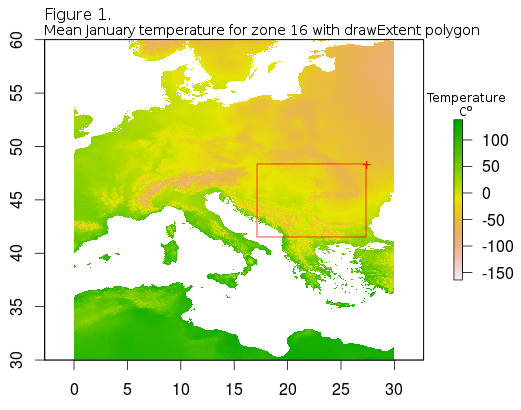After reading in the basic data, raster package offers several formats in which to keep the data, besides the basic raster. The most useful one is a raster stack which really is just the piled-up rasters that can be manipulated together. Also one useful visual help is the addition of the country boundaries followed by zooming in to the extent of the country or region where the samples originate from. Country boundaries .shp files is freely avalilable from thematicmapping. When downloaded they should be in the same directory as the GeoTiffs.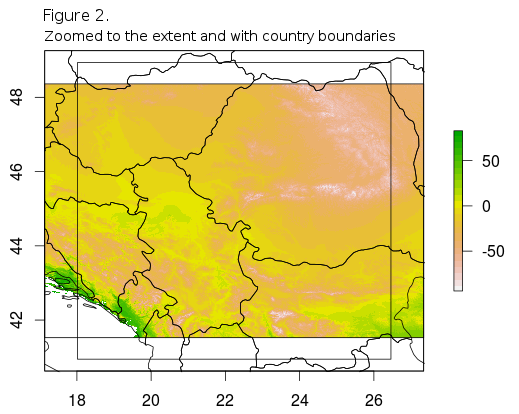Before final extraction of the climate data it can be useful to plot each month`s mean temperature for the selected extent of the rasater. This can be easily achieved by using the rasterVis package levelplot and the extentR variable, which can be used to cut through all raster layers in the stack.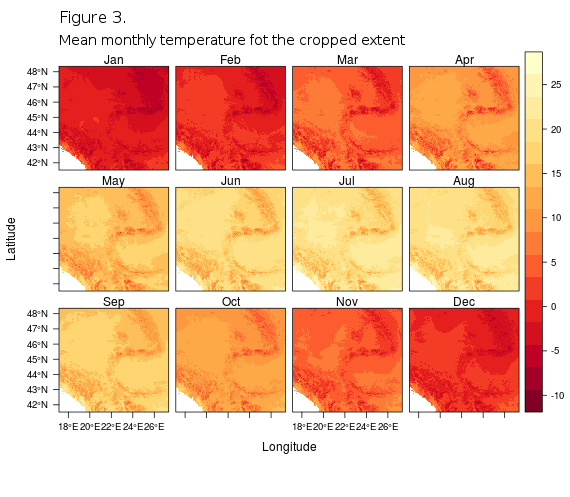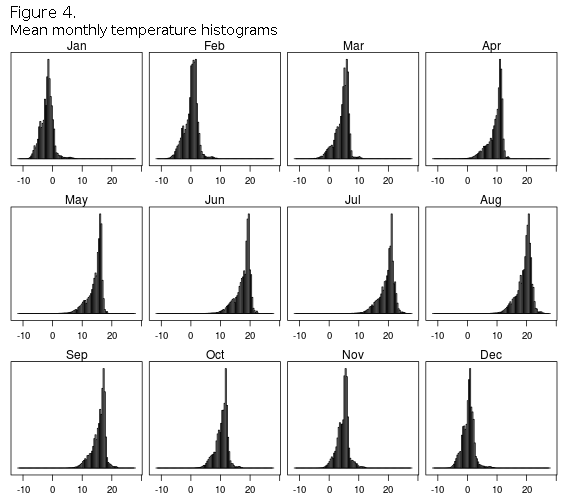Slightly more efficient than the drawExtent is the spatialPolygons function from the sp package that will be used for definition of the real sampling localities, by simply drawing boundaries of the localities by hand or using the known positional data. It is best if approximate latitude/longitude coordinates are known in advance so that the defining polygon can be drawn connecting several corners-points, that form the broader sampling area border. If coordinates are not known in advance then the drawExtent should be used first for finding the latitude/longitude of the spatial polygon points, and then making the SpatialPolygon object out of them, as described next.

After all spatial polygons are defined, final step involves the extraction of the mean temperature values from the points encircled by the polygon in question, for all months. Since GeoTiffs are raster formats, they are carrying the information on the temperature in the points of the bitmap grid, so if the polygon is too small, small will be the number of the grid-points inside, maybe smaller than the number of individuals. To avoid this make sampling area broader, and in this case polyTara has some 220 individual grid points inside, which means 220 values for the mean temperature in the area. If we have i.e. ten individuals per population it is necessery to have also 10 mean temperature values, so that both blocks in subsequent PLS would have same dimensionality. R`s basic sample function can be used to extract random values from the i.e. 220 points within the population (Tara and R1) polygons. This simulates random sampling of individuals from the sampling locality and after that any analysis can be done, from linear models to PLS, which will be shown in future posts.

Figure 5. shows the locations of tara and R1 spatial polygons within the zoomed extent of the zone 16, while in Figure 6. barplots are shown for 10 random points for all months, and for both localities, for comparison.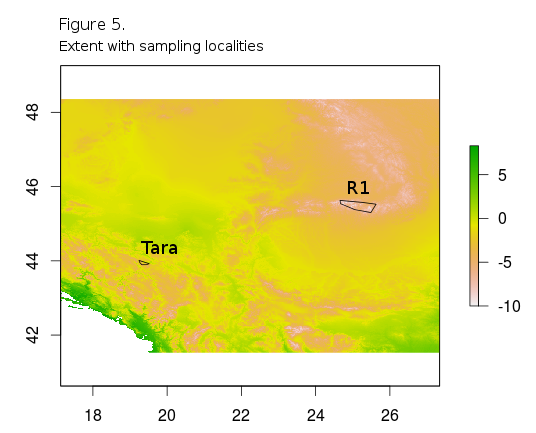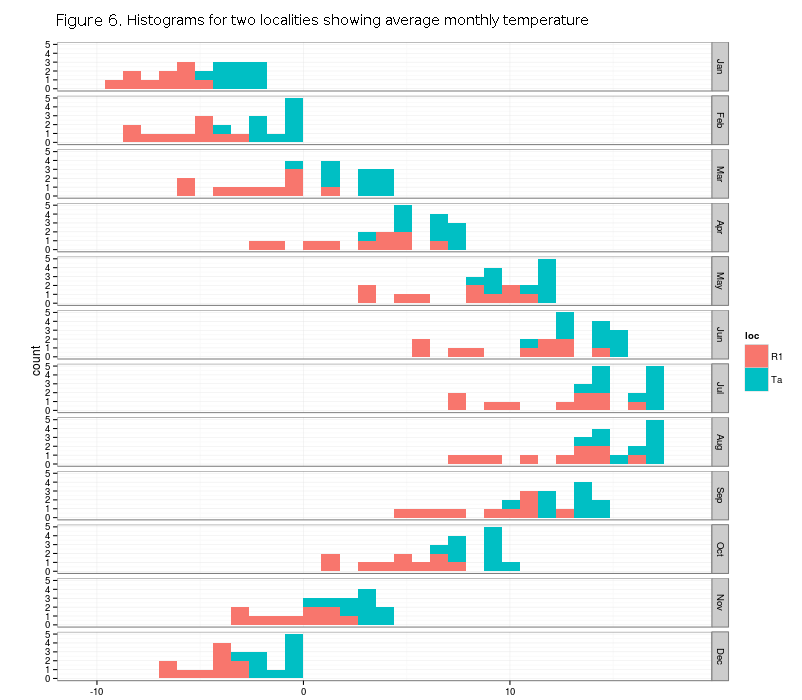It is obvious that the Tara locality has higer mean monthly temperature, on average for every month, and also it has less temperature variaton than R1.

]]>
<![CDATA[Python Forcing Monsters` Shape]]> 2014-03-08T11:49:21+01:00 http://paulidealiste.github.io/blog/2014/03/08/python-forcing-monsters`-shape Continuing on one of the previous posts about data generation in python, next natural step in the analytic procedure is the Procrustes superimposition. Since this procedure enables direct analyses of configurations` shape, and all subsequent explorative visualizations, it must be employed first. This post concerns with the basic superimposition procedure, involving only two landmark configurations, i.e. two generated monsters. One monster will be used as a reference and one as a target, which should undergo superimposition. First steps in data genration are the same as before, with the exception of polarRotator function that can take any numpy array and reorder it according to polar rotation angle. This is very useful since generated landmarks are not ordered properly and do not “feel natural” in plots and analyses.

The plot of configurations reveals their spatial relationship, as well as the general mean shape. This time, since landmarks are ordered properly, one line would be enough for representing mean shapes, and shapes of respective configurations.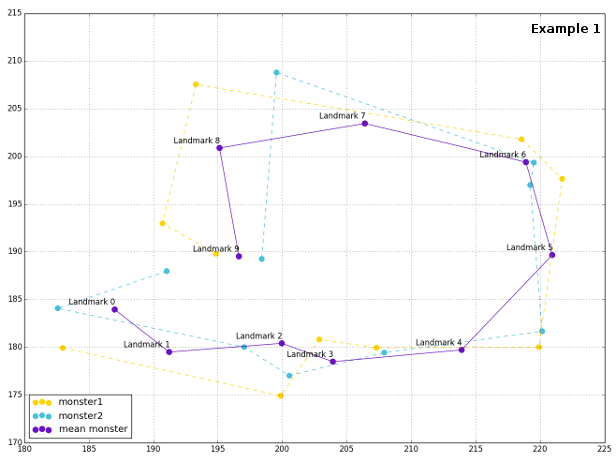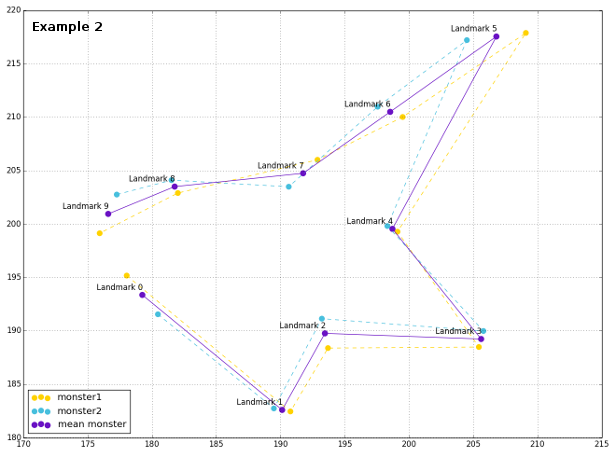Procrustes superimposition revolves around three features of shape extraction, that is invariance of landmark configurations to position, scale and rotation. There are a number of excelent textbooks about the mathematics and logic, as well as procedures for Procrustes superimposition (Bookstein, 19911, Dryden and Mardia, 19982, Zelditch et al., 20123), but for this post direct inspiration was Morphometrics in R (Claude, 2008), especially with the basic procedure presented in the following function definition.

Plot of the superimposed configurations reveals that the Procrustes python was really able to force monsters` shape be more similar, removing the effects of orientation, size and rotation.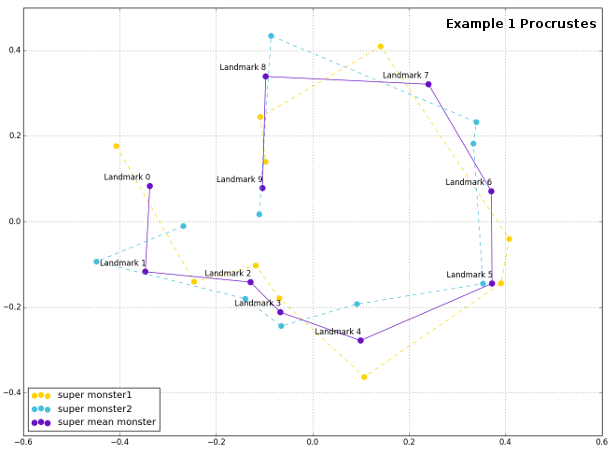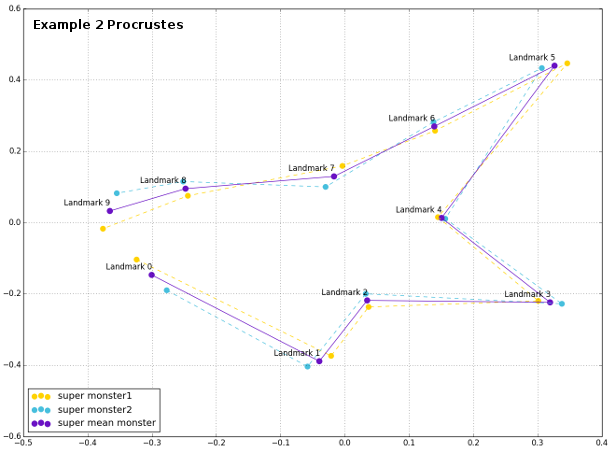Following posts should continue on this one and describe how the partial Procrustes superimposition for multilple configurations can be performed with fabulous sientific python.

1. Bookstein, F.L. 1991. Morphometric tools for landmark data: Geometry and Biology. Cambridge University press, Cambridge, UK.

2. Dryden, I.E. and K.V. Mardia. 1998. Statistical shape analysis. Wiley, Chichester, UK.

3. Zelditch, M.L., Swidersk, D.L. and H.D. Sheets. 2012. Geometric morphometrics for biologists: A primer. Second edition, Elsevier.

]]>
<![CDATA[R Generator and a Colorful PCA]]> 2014-02-23T11:24:16+01:00 http://paulidealiste.github.io/blog/2014/02/23/r-generator-and-a-colorful-pca As simple as it may seem, sample data generation is not a trivial task, especially when random landmarks are to be generated. Usually, one would use multivariate normal distribution-based generator (like mvrnorm in R`s MASS package) in order to generate correlated data. The following function was used to generate data similar to the real world datased, unfortunately based directly on it, by using the coefficients from regressions between successive columns in a data matrix, which represent landmark coordinates in XY data matrix. The following function uses this data matrix (446 individuals and 28 landmarks), and performs regressions between X-Y pairs for all coordinates. Finally it uses intercepts and slopes to infer mean and SD for rnorm function, random number generator.

When the function finishes the output is also an XY matrix, which needs to be converted to an array in order to use it in gpagen function from the geomorph package. After that the procedure follows all the usual steps of the GM analysis, with the exception of factor levels generation in order to simulate grouping, and finally performing PCA on the Procrustes shape variables.

PCA is then done using the usual R`s prcomp function and ggplot2 for plotting the data points using fantastic ColorBrewer color schemes (which are the names of types and palettes in ggplot2 scale_color_brewer geom). In order to fine-tune the PCA figure, the ggplot2 can also use custom fonts for plot annotation. Prior to that, fonts must be imported and registered, which is greatly facilitated by using the extrafont library.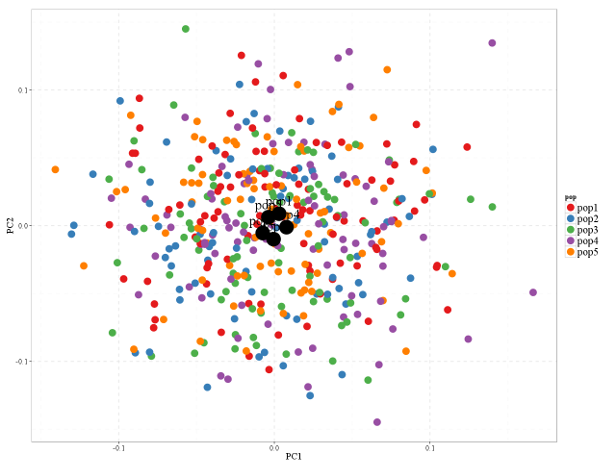The plot indicates very little differentiation between the populations, but I guess that`s well expected since so much randomness is at hand.

]]>
<![CDATA[XY Outlines in GIMP and imageJ]]> 2014-02-01T11:23:55+01:00 http://paulidealiste.github.io/blog/2014/02/01/xy-outlines-in-gimp-and-imagej This post continues on the first post about outline deformations and presents a simple way for generating outlines as XY data. XY format is very convenient for representing outline and line art in R or python. This type of visualization can be produced using GIMP and imageJ through a sequence of easy steps. GIMP enables easy extraction of the central object from the image background. First step in outline extraction should be the precise definition of the object boundaries, such that the contrast between the object and the background is maximal. Example picture for this post is available here. After opening the picture in GIMP workspace it looks like in Figure 1.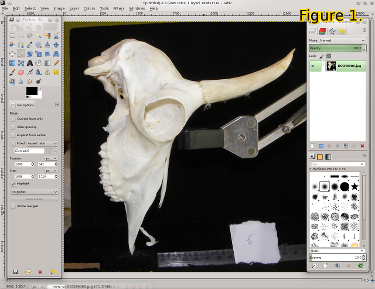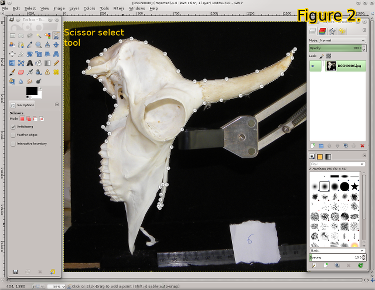The easiest way to separate this chamois cranium from its background is to use `Scissor Select Tool` from the GIMP toolbox. This tool can nicely track the path between sucessive control points that should be placed along the desired object (Figure 2). When control points are placed along the shape, selection can be completed by pressing enter, and the background can be deleted by inverting selection pressing `Ctrl+I`. and deleting it using the delete key (Figure 3). Selection should be inverted once more and converted to path; from the `Select` menu `To Path`. Finally, stroke path (Figure 4, path card and right click on selection, `Stroke path`) gives the desired outline that can be exported as .tiff for imageJ through `Export` in the `File` menu.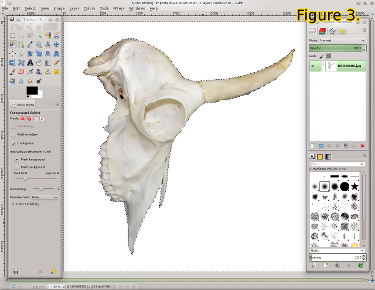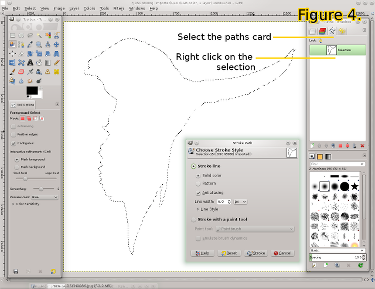In imageJ, picture should be converted to binary in `Process` menu, by selecting `Binary` and `Make Binary`. Final step is saving the binary image selection as XY data. First the outline should be selected by the magic wand selection tool (Figure 5) and then the image should be saved in XY format, in `File` menu, `Save as` and select `XY coordinates`. The XY data is also available here.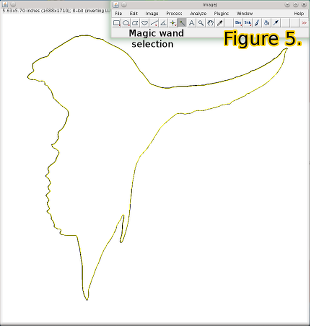Now the .txt file could be easily imported into R and python.

]]>
<![CDATA[Random Landmark Monsters in Python]]> 2014-01-17T11:36:06+01:00 http://paulidealiste.github.io/blog/2014/01/17/simple-random-landmarks-in-python Generating random datasets is facilitated by powerful algorithms in both R and python that are capable of drawing random numbers from predefined distributions. For simulating geometric morphometric data the only important constraint is correlation between x and y coordinates of landmarks. Spacing of landmarks in also important, especially if the aim is to generte life-like data set, but it is not essential (hopefully) for morphometric analyses to work. Python scipy stack (numpy, scipy, pandas and matplotlib + Ipython console) is a great budnle which enables both random number generators and great visualizations. The code presented in this post uses all of these libraries, especially pandas, which offer the basic data structure used for storing landmark data, DataFrame. Also, it can just be pasted into Ipython console window (afer installing any of the scipy stack python distributions). The basic idea is to use uniform distribution random number generator (line 7) in order to generate ranges for the spacing of landmark groups, and then multivariate normal random distribution for creating the two-column correlated data (x, y coordinates, line 8). This code will simulate the situation with 10 landmarks recorded on 200 individuals. Spacing of landmarks can be controlled by the ranges of uniform generators (175-220 in code), and the spread of landmarks is contolled by input covariance matrix for multivariate normal generators ([[3,0],[0,3]] in code).

allCoords contain all generated landmarks, while meanCoords is the collection of mean coordinates for each landmark. In order to visualize the generated data, a convenient “outline” can be drawn, connecting all landmarks smoothly. Since generated landmarks are not ordered in a way that permits simple connect-the-dots line between them, they should be rotated, and ordered differently. This can be done by using the centroid coordinates (from all landmarks) and the polar angle between the lines connecting centroid and each landmark. If polar angle is used, then landmarks (from meanCoords) can be ordered according to its value, clockwise. Polar angle can be calculated as the arctan between x and y coordinates of mean landmarks and the centroid.

If a path would be drawn between the mean landmarks now, it would be irregular, and not too informative. One way of constructing the smooth connection between landmarks is to use interpolation algorithms that are part of scipy.interpolate. One issue with this approach is the connection between the first and the last landmark, since it must be added to points DataFrame as eleventh landmark, with x,y coords the same as for the first one, in order to complete the path. This added segment behaves erratically during interpolation so the generated figures might be distorted. But this will not happen always and all program routines could be re-run as long as the desired, nice, result emerges.

Finally, plots are produced sequentially, first all landmarks, then mean landmarks, and finally the outline.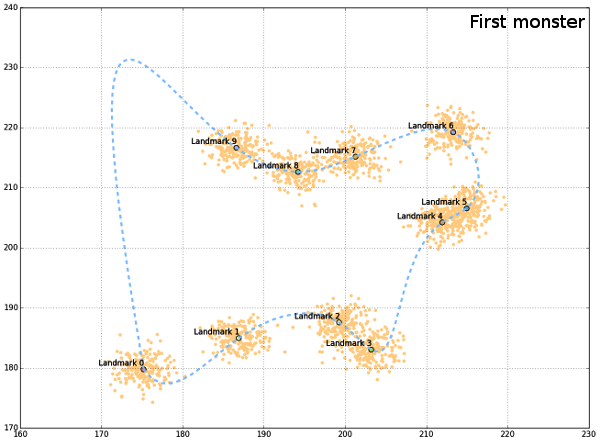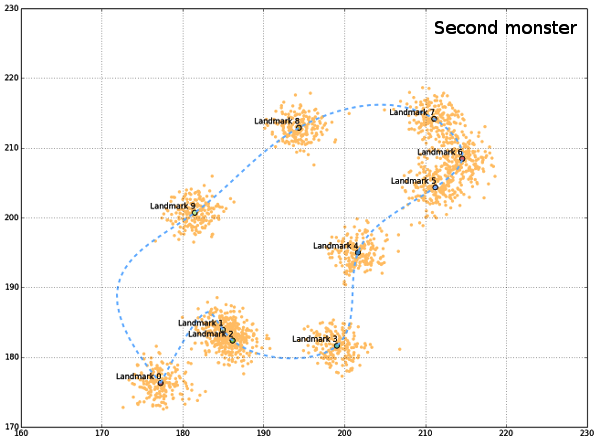Generated “monsters” are just there to get the idea of a possible shape, although the create the impression of the “outline”, such that all landmarks are sampled from the outer perimeter of the object. The code presented would not be complete if it wasn`t for the help from people from stackoverflow (here, here and here).

]]>
<![CDATA[Colorful Outlines for Shape Comparison]]> 2014-01-09T19:55:01+01:00 http://paulidealiste.github.io/blog/2014/01/09/colorful-outlines-for-shape-comparison This procedure is based on the outlines generated from the digital photos using imageJ and converting imageJ images to x-y continuous outline data available from here. Its goal is to present a visual overview of global shape differences between individuals from natural populations, using landmark data from ventral projection of their crania. All outlines are based on deformation via Thin Plate Splines, using mean shapes for populations as deformation targets and references. Superimposition methods as well as preliminary GM analyses were done in R and marvelous geomorph package by Dean Adams and Erik Otarola-Castillo. Additionally, since one of the common points of contemporary scientific research is the reproducibility of solutions offered all posts will also contain randomly generated sample data that could be used similarly to real-world datasets. Sample generation can be very useful, especially in teaching, so I intend to focus on it in future posts.

The workspace “capreolusRgen.RData” (which can be downloaded from here) contains several randomly generated datasetes of 657 individuals and 28 landmarks, named “capreolusSample#”. These data was generated on the basis of real-world values, using the linear regression model to control random number generators. The code that was used probably does not repoduce the sampling of landmarks well, especially regarding correlations between pairs of landmark coordinates or the landmarks that conform to the object symmetry, but for the purpose of illustration in this post, I hope they should be fine.

Following the Procrustes superimposition is the calculation of mean shapes, both for all males and for separate populations. After mean shapes are calculated the only thing left is to use TPS in order to deform outlines (variable d), using mean shape of all males as a reference and mean shape of populations as target. This can all be done using Morpho R-package from Stefan Schlager.

Finally, depicting shape changes can be achieved by wonderful Hadley Wickham`s ggplot2 R-package. This package has a neat way of “forcing” you to keep your data organized, so all variables are inside one data frame, both quantitative and qualitative.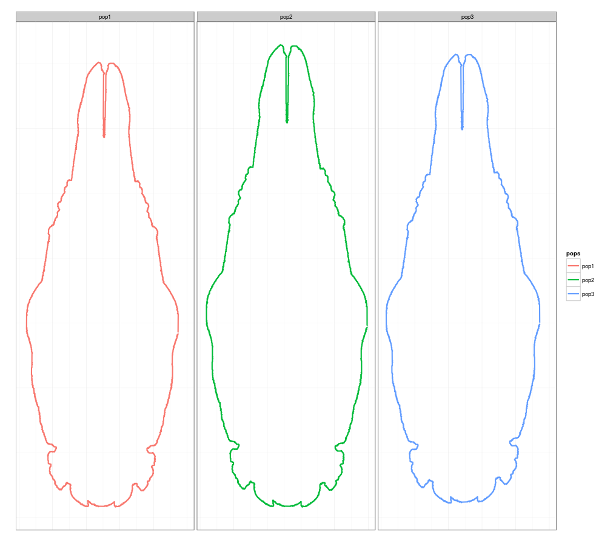By inspecting outlines it can be seen that the individuals from pop1 are the smallest while the ones from pop2 are the largest. Shape differences are also determined by the relationship of length to width, so that individuals from pop2 have the widest crania, while the ones from pop1 have the narrowest. Also, it can be seen that in the individuals with the largest crania, size differences are detemined mostly by dimensions of the anterior part, maxillary and rostral regions, that are both wider and longer with respect to individuals with smaller crania. Posterior part of the cranium is more similar between individuals from different populations, and it may be more stable.

]]>